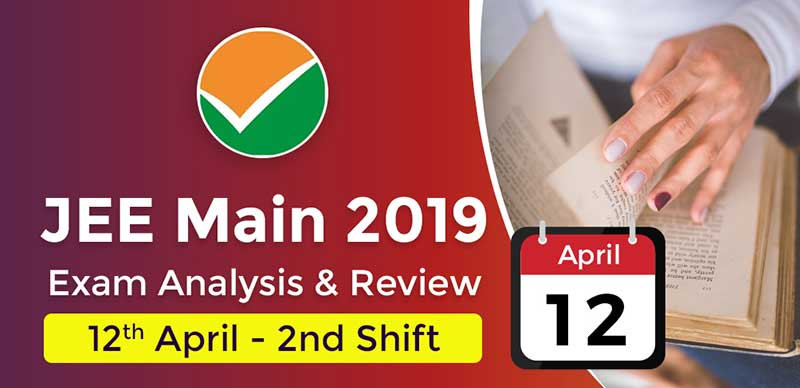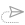### JEE Main 2019: Exam Analysis For Paper 1 (12th April - 2nd Shift)

The 2nd shift of JEE Main paper 1 has now ended for April 12, 2019. The analysis and reaction of students are provided here on this page.

The shift 1 paper of JEE Main 2019 was of moderate level as per the students. You can check JEE Main 2019 Shift 2 analysis for 12 April exam from below

Level of Difficulty in Each Section

 Level of Difficulty Subject Difficult Physics Moderate Mathematics Easy Chemistry

Subject wise analysis for JEE Main 12 April 2nd shift.

Analysis for Physics:

Physics was the toughest section in the second shift of April 12, 2019. It was more numerical based and hence came out to be lengthy.

Topics covered were tricky and not direct. There were questions from Mechanics, polaroids, optics. There were no questions from semiconductors.

Qs.1: There are two submarines A and B. Submarine A moves at 27 km/hr and gave a signal of 500 hertz. Submarine B returns at 18 km/hr. Calculate at which frequency returns for Submarine A

Qs.2: fcc lattice was given, find the distance between the two nearest voids.

Qs.3: A graph was given in which the rod is on the screw and it is rotating at a constant angular velocity. Plot another graph in the angular velocity to show the distance between the axis and the rod.

Qs.4: Find the distance of image of an object(s) placed on the surface of water from the surface a) 6.7 cm b) 13.8 cm c) 5.0 cm d) 2.5 cm

Ans : 6.7 cm

Qs.5: A projectile is thrown at an angle θ and with velocity v from the ground. If the equation of trajectory is y=2x-9x². Find the value of θ

Analysis for Chemistry:

Chemistry was easy for students. More weightage was given to organic and inorganic although physical chemistry was less in the paper.

There were less numerical based questions and more of reactions to be done.

Qs.1: Between Glucose and Galactose, whose configuration will be different?

Ans : C4

Qs.2: Which compound has maximum Oxidation potential?

Qs.3: Find the oxidation of Menthy benzene to Carboxylic acid

Qs.4: Components present in photochemical smog are? a) N2, O2 b) CO2, NO c) NO, O3 d) O3, N2

Ans : NO, O3

Qs.5: Which of the following is Thermosetting polymer? a) Nylon 6 b) Bakelite c) Polythene d) Polysterene

Ans : Bakelite

Qs.6: The group no, number of valence electrons and valency of an element with atomic number 15 respectively are a) 16, 6, 3 b) 16, 5, 2 c) 15, 5, 3 d) 15, 6, 2

Ans : 15, 5, 3

Qs.7: In face-centered cubic cell the distance between two tetrahedral voids is

Ans : √3a/2

Qs.8: Which element liberates H2 gas on treatment with both acid as well as base a) Mg b) Ca c) Zn d) Hg

Ans : Zn

Qs.9: Which is correct statement for N(SiH3)3 and N(CH3)3 a) N(SiH3)3 is pyramidal and less basic than N(CH3)3 b) N(SiH3)3 is tetrahedral more basic than N(CH3)3 c) N(SiH3)3 is pyramidal and more basic than N(CH3)3 d) N(SiH3)3 is tetrahedral and less basic than N(CH3)3

Qs.10: The correct order for thermal stability (1) MgCO3 > CaCO3 > SrCO3 > BaCO3 (2) BaCO3 > SrCO3 > CaCO3 > MgCO3 (3) SrCO3 > CaCO3 > MgCO3 > BaCO3 (4) CaCO3 > MgCO3 > SrCO3 > BaCO3

Ans : BaCO3 > SrCO3 > CaCO3 > MgCO3

Analysis for Mathematics:

Mathematics was lengthy in the second shift. Although, questions from ellipse and hyperbola were easy.

Also, questions from Permutations and probability were easy. Mathematical reasoning, boolean algebra and complex numbers were frequently repeated in all the shifts till date.

Qs.1: There are two circles, radius of one circle is 5 and the other is 12. The two circles are intersected by a line at 90 degrees. Find the common chord length?

Qs.2: Out of the 31 objects, select 10 objects. Note that 10 objects are same and 21 are different. Find out how many ways are there to select these object?

Qs.7: Number of solutions od the equation: 1+sin4x=cos23x in x ∈ [-3π/2, 3π/2] is a) 3 b)7 c) 4 d) 5

Ans : 3

Qs.8: If the compound statement p->(nq∨r) is false then the truth values of the statements p, q, r are a) TFF b) FFF c) TFT d) TTF

Ans : TTF

Student Reaction:

• There was a question in mathematics, there were two curves and the students had to find ist common tangent.
• There was 01 question from Complex number, 2 from Probability, 1 from Sequence and Series, 02 from Trigonometry in the Mathematics Section.
• There was 01 question from Mechanic, 2 from optics, 1 from Em waves, 03 from thermodynamics, 02 from wave optics.
• There was a question from Mathematics, in which the students had to find the common tangent for Hyperbola and Parabola.
• The question for probability was that a certain number of boys were given, the students had to find the number of girls.
• In matematics, there were questions from Eclipse, Circle, Probability.
• There was a question from Boolean Algebra in which the students had to find the negation of p and -q.
• In Chemistry, the candidates had to name the compound with less 2s orbital energy.
• There was a question in Physics in which it was given that there is a 2 watt sound source and it is at a distance and send sends the sound
• In Mathematics, there were questions from Sequence and Series, Integration and Area.
• One of the student commented that physics was more of Calculative Type and Maths was lengthy
• No repetition of questions from the January attempt.
• There was an equation based question in the Chemistry section in which Initial concentration was given and the students had to find final rate.
• In Physics, there was a question in which the students had to find the band frequency and modulation index.
• Organic chemistry had maximum weightage iin comparison to Physical chemistry.
• In Mathematics, there were maximum questions from 3-D
• In Physics there were questions from Sound waves, there were few questions from Optics as well.
• Chemistry was easy and mathematics was of Moderate
• Physics was lenthy and tricky, however, the difficulty level was moderate.
• Exam was easy in terms of difficulty level in comparison to January attempt16 October, 2021, 10:17 am##### JEE Advanced 2021 Result On This Date, Check Admission Process Here

11 October, 2021, 11:11 am##### JEE Main Paper 2 Result 2021 Declared; Direct Link Available Here

6 October, 2021, 10:46 am##### JEE Advanced 2021 : response sheet released, Full Details Inside

6 October, 2021, 10:22 am##### JEE Advanced Exam Analysis 2021 : Section Wise Memory Based Questions of Paper I & II Held On 03/10/2021

4 October, 2021, 10:15 am27 September, 2021, 10:51 amWe are providing something unique, useful and most importantly fun. By giving students a tool to find instant solutions to their doubts, we’re trying to make every student self-sufficient in practicing & completing their homework×